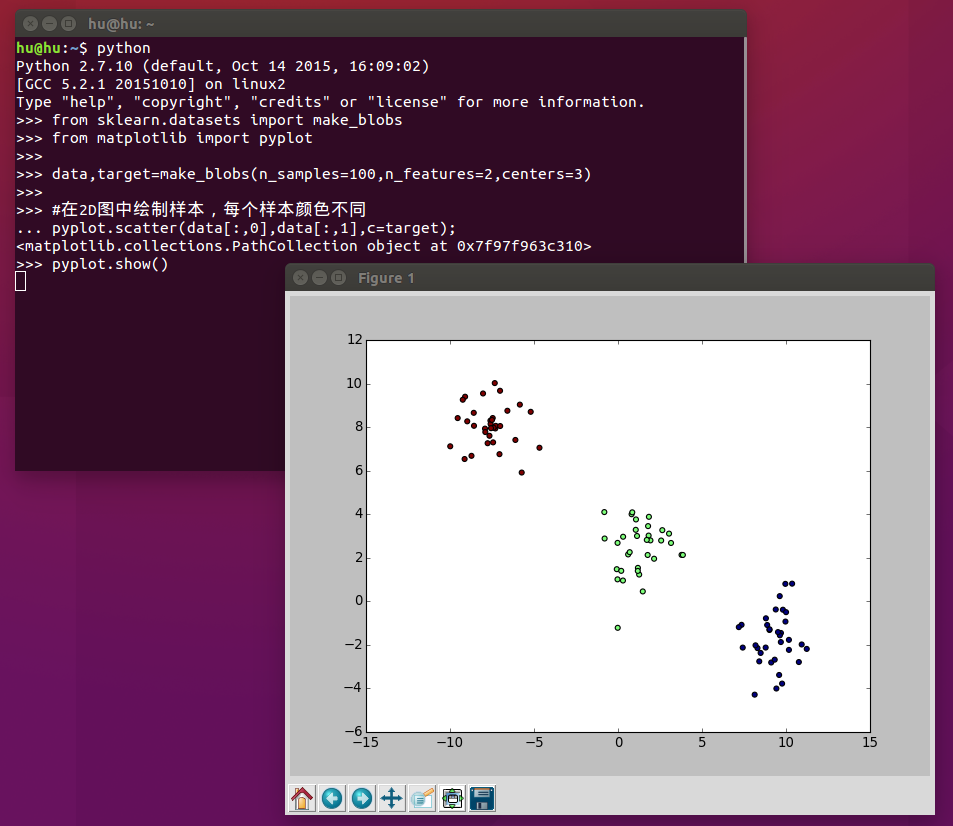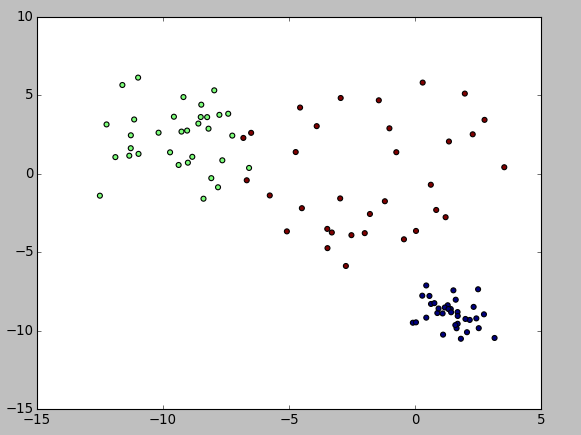# Python数据挖掘框架scikit数据集之make_blobs聚类数据生成器

scikit中的make_blobs方法常被用来生成聚类算法的测试数据，直观地说，make_blobs会根据用户指定的特征数量、中心点数量、范围等来生成几类数据，这些数据可用于测试聚类算法的效果。

make_blobs方法的API如下：

``````sklearn.datasets.make_blobs(n_samples=100, n_features=2, centers=3, cluster_std=1.0, center_box=(-10.0, 10.0), shuffle=True, random_state=None)[source]
``````

n_samples是待生成的样本的总数。

n_features是每个样本的特征数。

centers表示类别数。

cluster_std表示每个类别的方差，例如我们希望生成2类数据，其中一类比另一类具有更大的方差，可以将cluster_std设置为[1.0,3.0]。

``````from sklearn.datasets import make_blobs
from matplotlib import pyplot

data,target=make_blobs(n_samples=100,n_features=2,centers=3)

#在2D图中绘制样本，每个样本颜色不同
pyplot.scatter(data[:,0],data[:,1],c=target);
pyplot.show()
````````````from sklearn.datasets import make_blobs
from matplotlib import pyplot

data,target=make_blobs(n_samples=100,n_features=2,centers=3,cluster_std=[1.0,2.0,3.0])

#在2D图中绘制样本，每个样本颜色不同
pyplot.scatter(data[:,0],data[:,1],c=target);
pyplot.show()
``````#### You may also like...

##### 加QQ群下载完整Eclipse项目

WebCollector网络爬虫：250108697(已满)

WebCollector网络爬虫2群：345054141